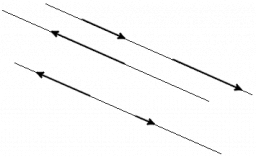# Coordinate 59833

Determine the unknown coordinate of the vector so that the vectors are collinear: e = (7, -2), f = (-2, f2)
c = (-3/7, c2), d = (- 4.0)

f2 =  0.5714
c2 =  0

### Step-by-step explanation:Did you find an error or inaccuracy? Feel free to write us. Thank you!

Tips to related online calculators
Check out our ratio calculator.
Our vector sum calculator can add two vectors given by their magnitudes and by included angle.
Do you want to convert length units?